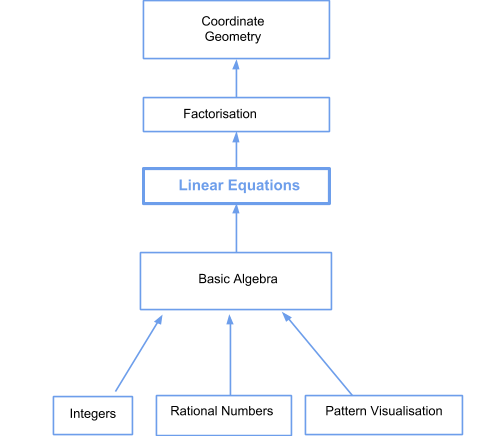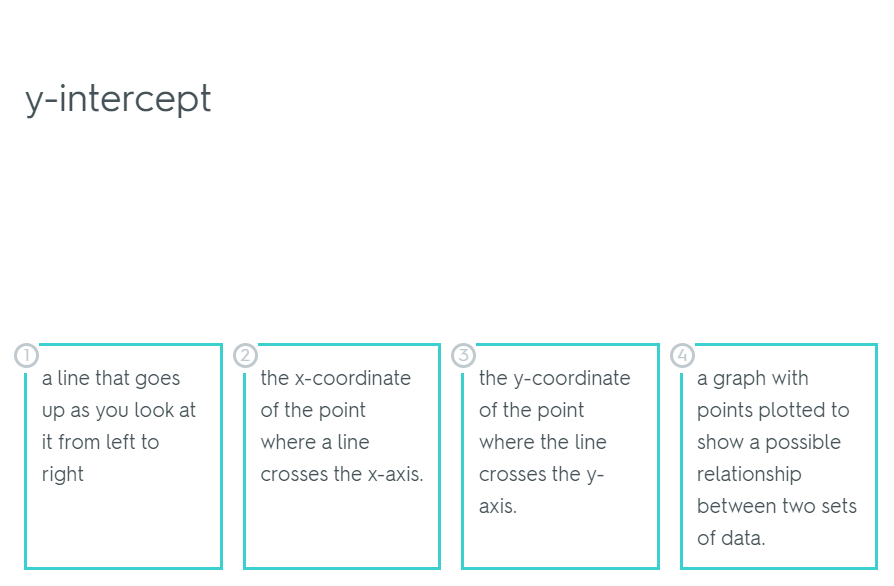In the verge of coronavirus pandemic, we are providing FREE access to our entire Online Curriculum to ensure Learning Doesn't STOP!

Go back to  'Maths'

## Introduction to Linear Equations

### Found ‘x’

Linear Equations are one of the topics students struggle with the most in Middle School. This is primarily because the topic is presented to students as isolated lines of text, devoid of any context## The Big Idea: What is a Linear Equation?

The very essence of a linear equation is that it represents an equation for a straight line

So, when you see an equation of the form:

y = mx + c

$${y}$$ and $${x}$$ are the variables, $${m}$$ is the slope and $${c}$$ is the $${y}$$ intercept (the point where the straight line cuts the $${y}$$-axis).

## How do I understand?

### The foundational nature of linear equations

Linear equations form a critical juncture in the mathematical journey of your child. After basic algebra, linear equations are the gateway to the whole world of abstraction that will allow your child to be fluent in the language of higher mathematics. Linear equations find their application in almost all walks of math, the most direct application being coordinate geometry. Have a look at the lattice below to see how pivotal a role linear equations play;### How can you visualise linear equations?

“Find x”. The most dreaded phrase that the everyday student comes across during their time as a middle schooler. Well, here’s some good news, it need not be anymore. Now, with the Cuemath way, your child gets the direction and context they need to fully internalize the concept of linear equations.

The key thing to be able to visualize is how an equation changes with the values of $${x}$$ and $${y}$$ and $${m}$$ and $${c}$$.

### Why linear equations? How are linear equations important?

Now that you are familiar with the basics of linear equation, it’s time for you to take a deeper dive into the concepts at play. You will be using linear equations to solve all kinds of problems, ranging from simple algebra to calculus.

## Sub Topics

However, before you dive in to the deep end of the pool, it’s best to familiarize yourself with the basics. Check out the links below to find out everything that Cuemath has to offer on the topic of Linear and Quadratic equations. Below topics cover both concepts and worksheets:

## How do you teach linear equations?

Linear equations are one of the most crucial tools in your child’s math arsenal. So, it’s imperative that they are set in order and can be wielded at a moment's notice. Here are a few things that you can do to ensure that your child attains a mastery of linear equations.

### Break it down:

Like all concepts in mathematics, linear equations don’t exist in isolation. In order for your child to have crystal clear concepts, ensure that they regularly brush up foundational concepts that lead up to linear equations. This process is called Chunking

It always helps to keep freshening up on concepts of basic algebra and operations so that your child’s thought process is more focused when doing linear equations. A very good way to practice and ensure chunking is to incorporate questions from basic algebra and operations in between questions for linear equations. This way you child is always focused and ready.

### Flash Cards are your friends:

Flash cards are excellent learning tools. They keep you on your toes and can be pulled out at a moment’s notice for a quick dose of revision. Repeated practice and use of such flash cards keep concepts fresh in the mind of your child and ensure long term retention.### Practice makes perfect:

After understanding the concept comes practice. Nothing helps children learn faster than applying the concepts that they are taught. Research has shown that it allows them to cement their concepts and it leads to longer retention.

Linear equations is a topic that raises a lot of questions in young minds, especially while starting out. It is very important to nip these issues in the bud and not let them linger on and grow. Here are a few of the most common doubts and issues that kids face while studying linear equations.

Transposition: Solving linear equations hinges on grouping like variables and constants together and then solving for each. Let’s take the simple example of a linear equation in one variable to illustrate the point.

3(x+1) + x = 5(x-2) + 12
⇒ 3x + 3 + x = 5x - 10 +12
⇒ 4x + 3 = 5x + 2

Now, in class, most children are told that transpose the constants to one side of the equal to sign and the variables to the other, and when you transpose, “change the sign”. However, no one tells you, why. Well, here’s the “Why behind the what”

The thing with “transposing” constants and variables is that you aren’t “moving the digits across the equal to sign”, what you’re actually doing is adding, subtracting, multiplying or dividing the same quantity from both sides of the equation. So taking the simplified form of the above equation:

4x + 3 = 5x + 2
Subtracting (5x + 3) from both sides of the equation
4x + 3 - (5x + 3) = 5x + 2 - (5x + 3)
⇒ 4x - 5x = 2 - 3
This is why it’s said that the signs are reversed when you group terms across the equal to sign.
⇒ -x = -1
⇒ x = 1